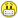+0

# CPhill's Puzzle For The Day.......!!!!

+3
1178
8

Can anyone think of 5 positive integers, a,b,c,d and e such that

a^2 + b^2 + c^2 + d^2 = e^2   ?????

[ There is more than one correct answer ]  !!!!!!

(Also....and I should have been more specific....the integers are all different ) !!!!Oct 8, 2014

#4
+28

Hm...

a = 4, b = 8, c = 10, b = 12, and e = 18 :)

Oct 10, 2014

#1
0

is the answer 1Oct 8, 2014
#2
+5

a = b = c = d = n;  e = 2n;  where n is any positive integer.

Oct 8, 2014
#3
0

Chris said all the integers were different!Oct 9, 2014
#4
+28

Hm...

a = 4, b = 8, c = 10, b = 12, and e = 18 :)

kitty<3 Oct 10, 2014
#5
0

Ding!!  Ding!! Ding!!!......we have a winner!!

kitty<3  !!!!

I came up with........

1 , 2 , 8, 10, 13

Note, that any positive integer multiples of these answers "work," too. (Just like in the Pythagorean "Multiples")

[Mmmm...I wonder if there is a general "formula" for generating the "correct" values??? ]Oct 10, 2014
#6
+5

Here's a way of generating more sets of values:

Choose a Pythagorean triple, say 3, 4, 5.  Let a = 3m, b = 4m, c = 3n, d = 4n, and e = 5√(m2 + n2

a2 + b2 + c2 + d2 = (32 + 42)m2 + (32 + 42)n2 = (32 + 42)(m2 + n2) = 52(m2 + n2) = e2

Now choose m and n to be part of another Pythagorean triple, say m = 5 and n = 12, and we have e as an integer (=5*13).

So a = 3*5 = 15;  b = 4*5 = 20;  c = 3*12 = 36;  d = 4*12 = 48; e = 5*13 = 65;

\$\${{\mathtt{15}}}^{{\mathtt{2}}}{\mathtt{\,\small\textbf+\,}}{{\mathtt{20}}}^{{\mathtt{2}}}{\mathtt{\,\small\textbf+\,}}{{\mathtt{36}}}^{{\mathtt{2}}}{\mathtt{\,\small\textbf+\,}}{{\mathtt{48}}}^{{\mathtt{2}}} = {\mathtt{4\,225}}\$\$

\$\${{\mathtt{65}}}^{{\mathtt{2}}} = {\mathtt{4\,225}}\$\$

(I don't remember seeing Chris's last line when I gave my previous answer.  Hmm!)

.

Oct 10, 2014
#7
0

Very nice, Alan.....I added that last line after you posted your first answer.....I meant for the all the digits to be different.......Oct 10, 2014
#8
0

Well Alan, I shall, give you 3 points for your first answer then - otherwise you would be getting cheated!Oct 11, 2014